# Class 12 Electrostatics

4649

Physics Electrostatics Level: Misc Level

How much energy is stored by the electric field between two square plates 8.0 cm on a side, separated by a 1.5 mm air gap, the charge on the plate are equal and opposite and of magnitude 420 u C.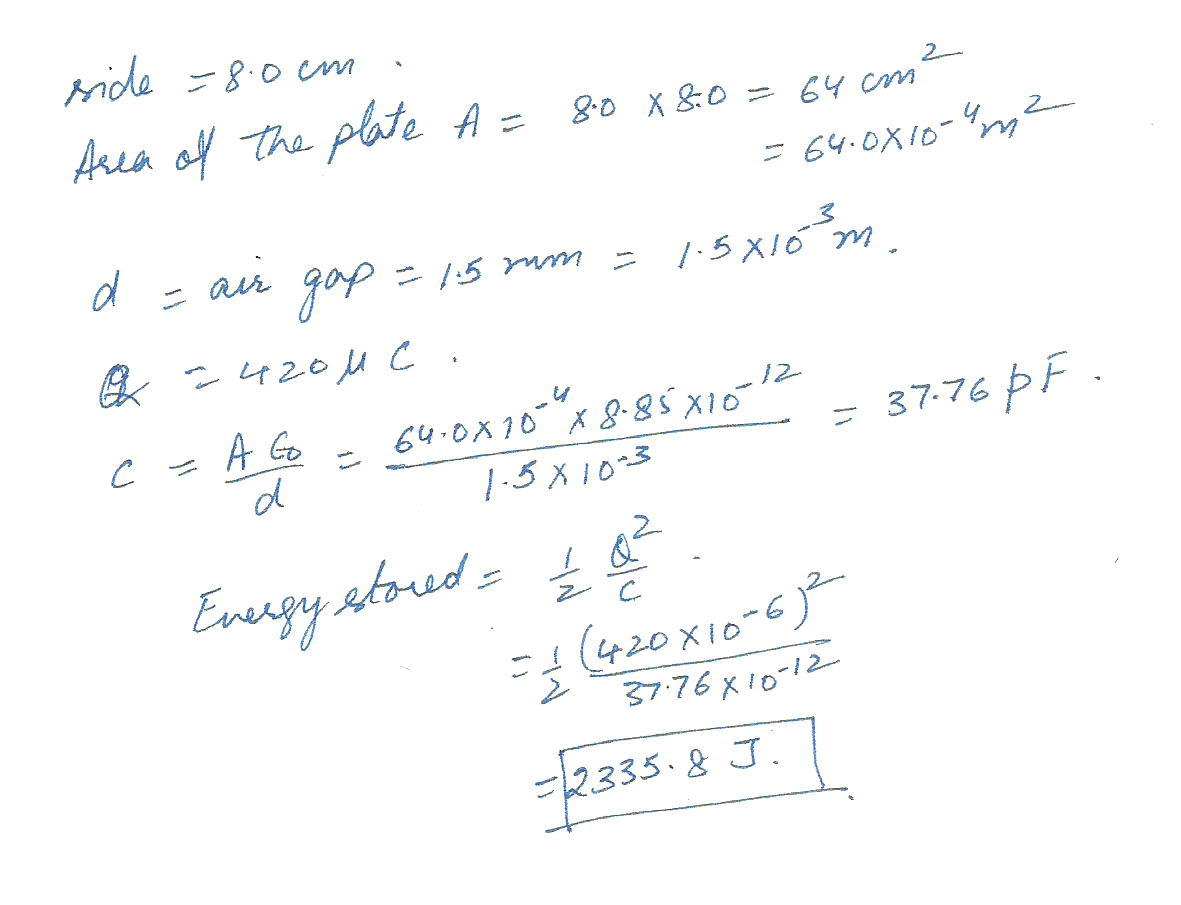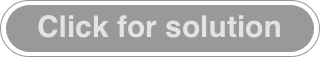4643

Physics Electrostatics Level: Misc Level

a point charge q create an electric potential of + 125 v at a distance of 15 cm. what is q ?4552

Physics Electrostatics Level: Misc Level

A pair of charged plates is used to accelerate singly ionized aluminum to 1250 m/s. The plates are 0.10 m apart. What is the voltage across the plates?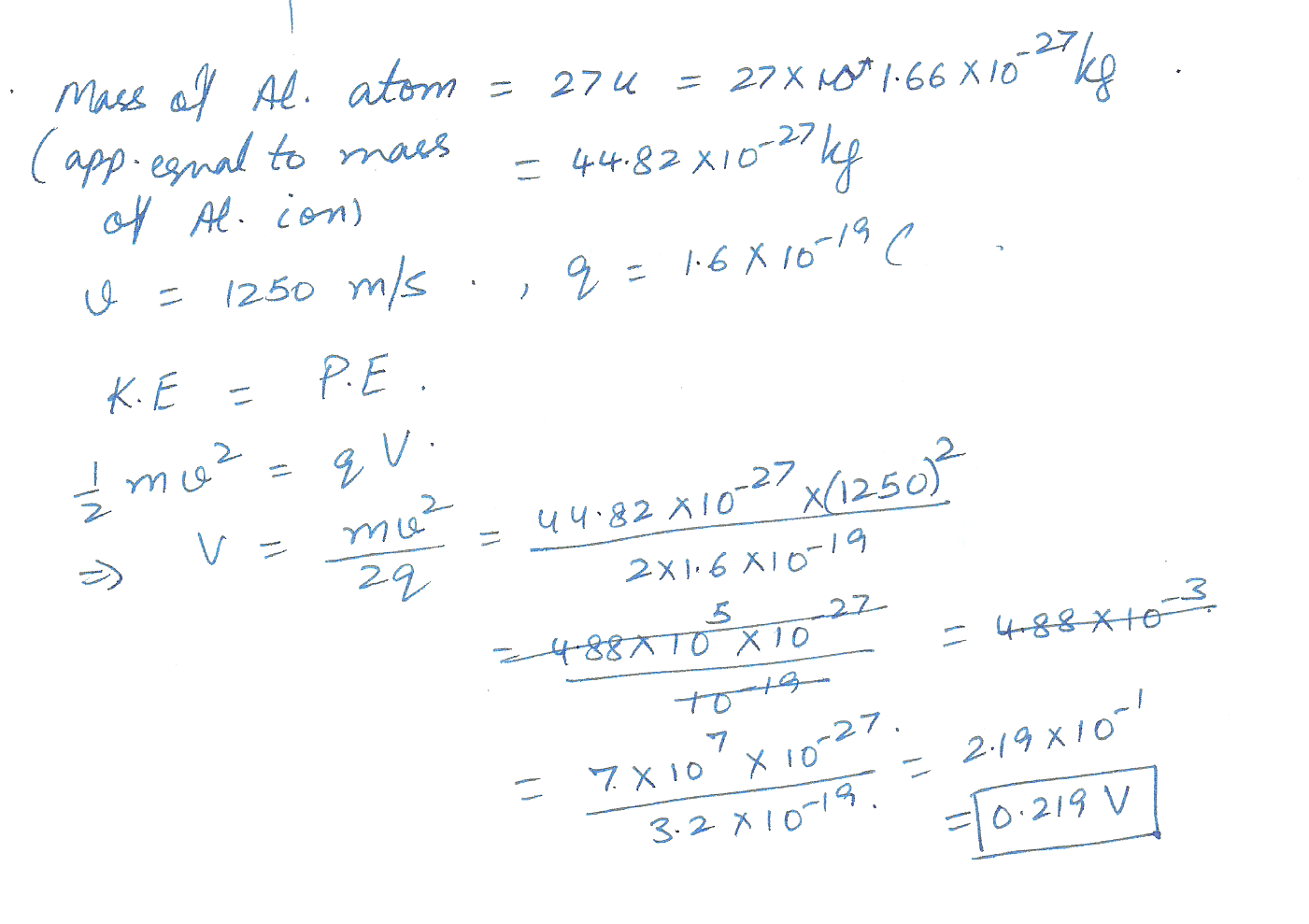4320

Physics Electrostatics Level: Misc Level

A thin spherical conducting shell has an inner radius of 6.8 cm and an outer radius of 7.0 cm. The shell carries a net charge of + 5 p C which results in a surface charge density magnitude on the inner surface of ( Hint: What do you know about the electric field due to a conductor? also find the surface charge density on the outter surface.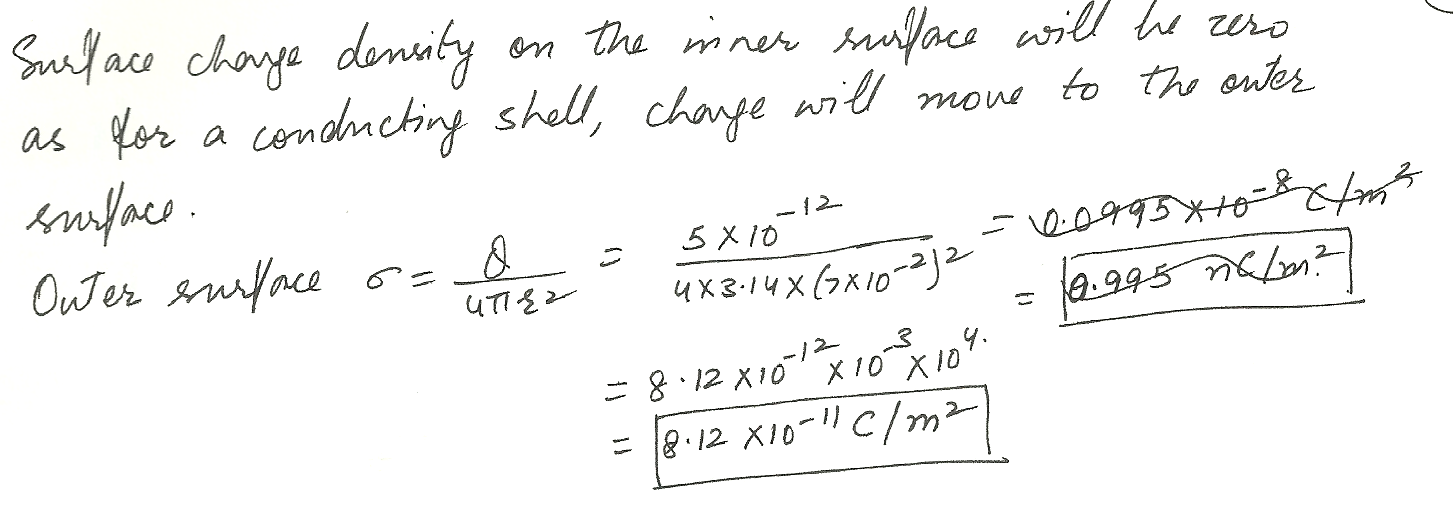4319

Physics Electrostatics Level: Misc Level

Two infinite parallel surfaces carry uniform charge densities of 0.20 n C/ m 2 and - 0. 60 n C /m 2. What is the magnitude of the electric field at a point between the two surfaces? (Assume the planes are nonconducting. It may help to draw a sketch showing the electric field lines between the planes.)4318

Physics Electrostatics Level: Misc Level

A charge of uniform surface density 0.20 n C /M2 is distributed over the entire xy - plane. What is the magnitude of the electric field at any point having z = 2.0 m? ( Assume the plane is nonconducting. )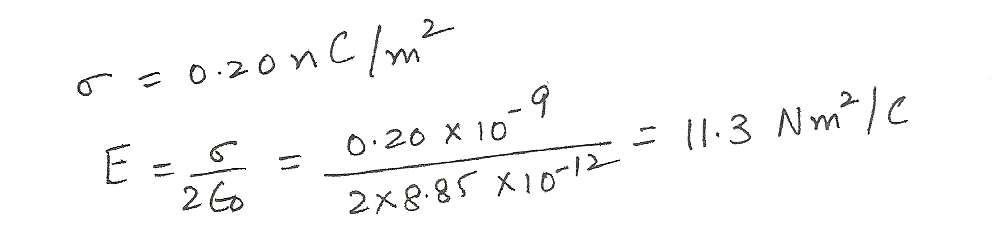4317

Physics Electrostatics Level: Misc Level

A charge of 0.80 n C is placed at the center of a cube that measures 4.0 m along each edge. What is the electric flux through one face of the cube?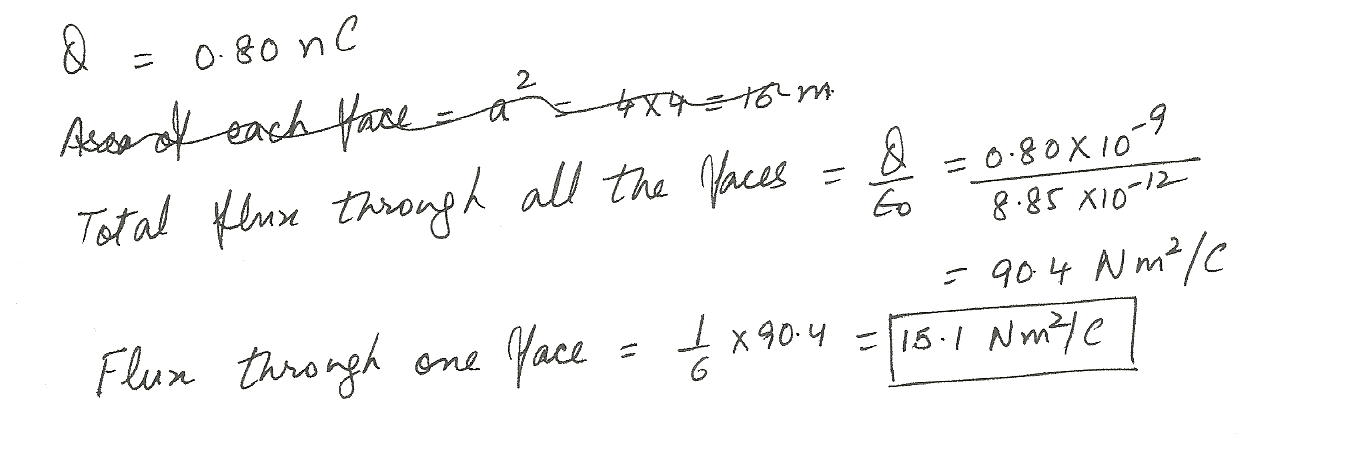4316

Physics Electrostatics Level: Misc Level

A point charge + Q is located on the x -axis at x = a, and a second point charge - Q is located on the x - axis at x = - a. A Gaussian spherical surface with radius r = 2 a is centered at the origin. The flux through this Gaussian surface is

a. zero because the net charge enclosed by the Gaussian surface is zero

b. greater then zero

c. zero because at every point on the surface the electric field has no component perpendicular to the surface

d.zero because the electric field is zero at every point on the surface

e.none of the above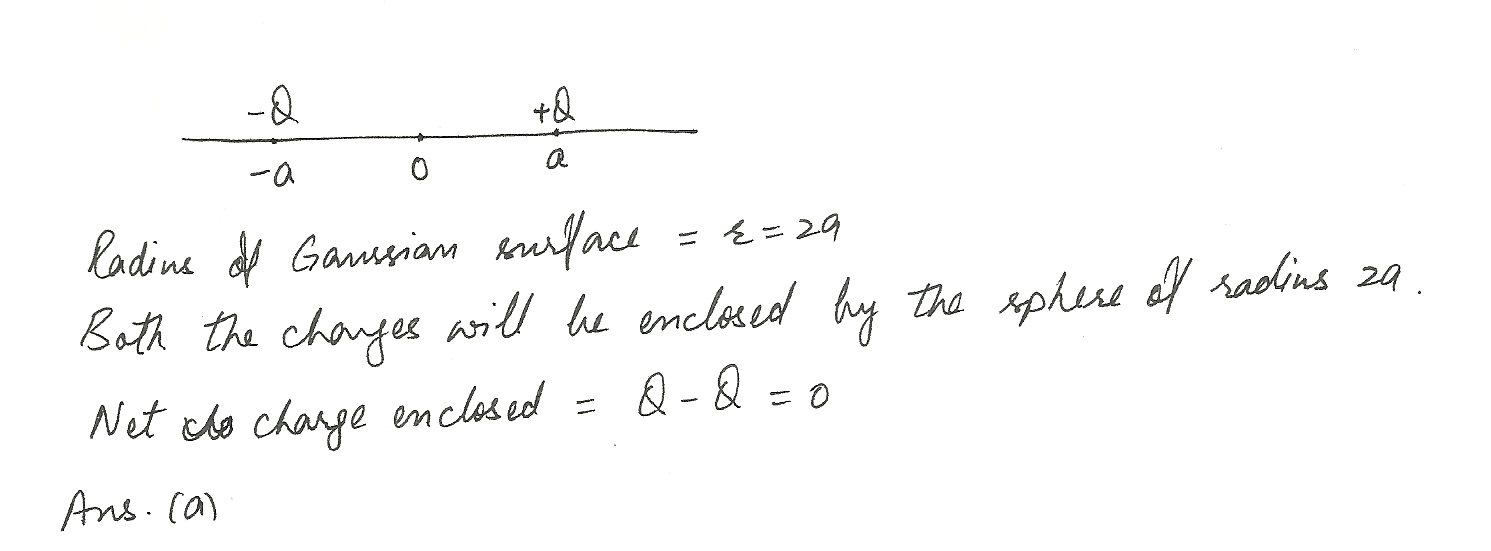4315

Physics Electrostatics Level: Misc Level

A uniform linear charge density of 4.0 n C /m is distributed along the entire x - axis. Consider a spherical ( radius = 5.0 cm ) surface centered on the origin. Determine the electric flux for this surface.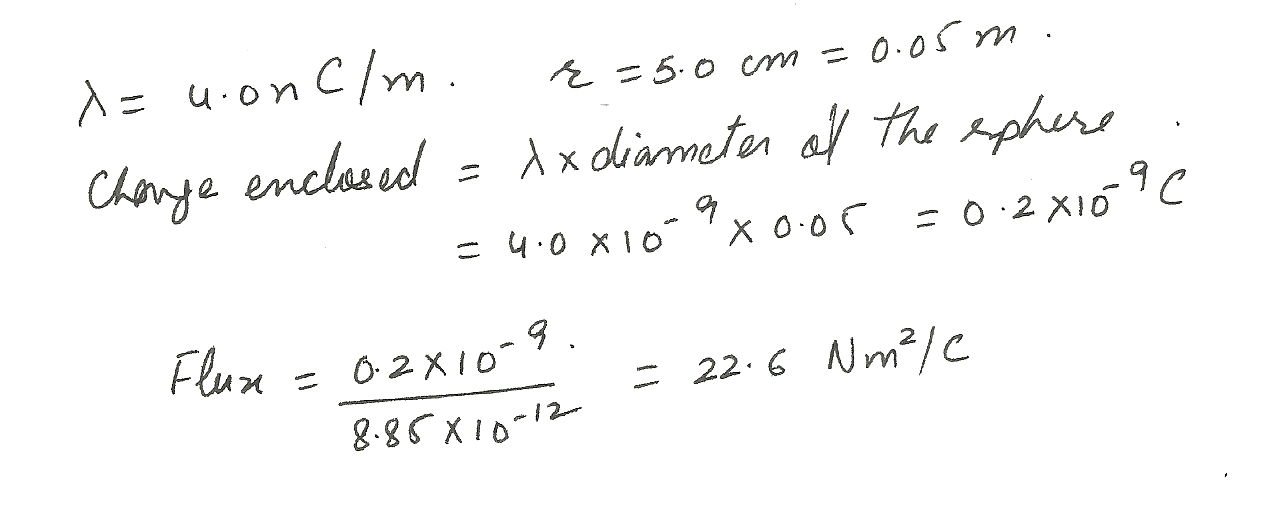4313

Physics Electrostatics Level: Misc Level

Two charges of 15 pC and -40 p C are inside a cube with sides that are 0.40 m in length. Determine the net electric flux for the surface of the cube.

(Note:1 p C= 1 x 10 -12 C. )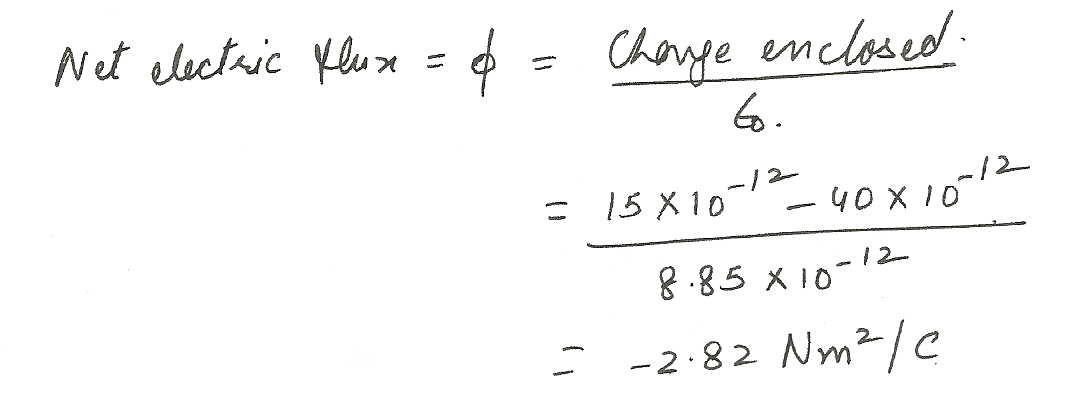Displaying 1-10 of 45 results.